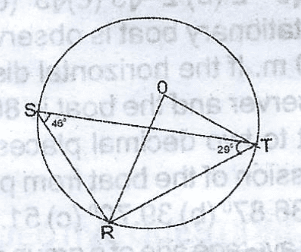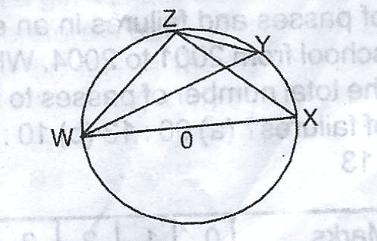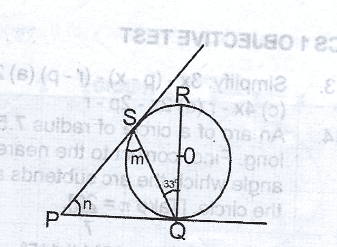# Mathematics 2017 Past Questions | WAEC

Study the following past questions and answers for JAMBWAEC  NECO and Post-JAMB. Get prepared with official past questions and answers for upcoming examinations.

31.  Given that t = $$2 ^{-x}$$, find $$2 ^{x + 1}$$ in terms of t.

• A. $$\frac{2}{t}$$
• B. $$\frac{t}{2}$$
• C. $$\frac{1}{2t}$$
• D. t

32.  Two bottles are drawn with replacement from a crate containing 8 coke, 12 and 4 sprite bottles. What is the probability that the first is coke and the second is not coke?

• A. $$\frac{1}{12}$$
• B. $$\frac{1}{6}$$
• C. $$\frac{2}{9}$$
• D. $$\frac{3}{8}$$

33. If the simple interest on a certain amount of money saved in a bank for 5 years at 212$\frac{1}{2}$% annum is N500.00, calculate the total amount due after 6 years at the same rate

• A. N2,500.00
• B. N2,600.00
• C. N4,500.00
• D. N4,600.00

34. Calculate the variance of 2, 3, 3, 4, 5, 5, 5, 7, 7 and 9

• A. 2.2
• B. 3.4
• D. 4.0
• D. 4.2

35. A circular pond of radius 4m has a path of width 2.5m round it. Find, correct to two decimal places, the area of the path. [Take22/7]

• A. 7.83m2${m}^{2}$
• B. 32.29m2${m}^{2}$
• C. 50.29m2${m}^{2}$
• D. 82.50m2

36.Fig. 1 and Fig. 2 are the addition and multiplication tables respectively in modulo 5. Use these tables to solve the equation (n 4$\oplus 4$)

• A. 1
• B. 2
• C. 3
• D. 4

37.The diagram shows a circle centre O. if <STR = 29 and <RST = 450, calculate the value of <STO

• A. 12º
• B. 15º
• C. 29º
• D. 34º

38.In the diagram, XY is a straight line. <POX = <POQ and <ROY = <QOR. Find the value of <POQ + <ROY.

• A. 60º
• B. 90º
• C. 100º
• D. 120º

39The diagram shows a circle O. If < ZYW = 33º

, find < ZWX

• A. 33º
• B. 57º
• C. 90º
• D. 100º

40.In the diagram, PQ and PS are tangents to the circle O. If PSQ = m, <SPQ = n and <SQR = 33º, find the value of (m + n)

• A. 103º
• B. 123º
• C. 133º
• D. 143º
Subscribe
Notify ofInline Feedbacks